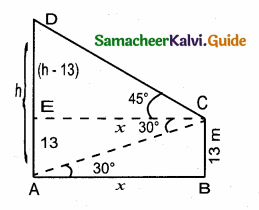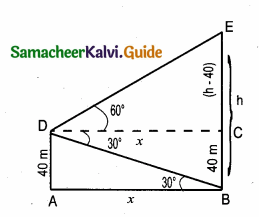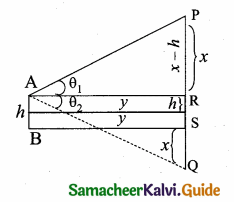Students can download Maths Chapter 6 Trigonometry Ex 6.4 Questions and Answers, Notes, Samacheer Kalvi 10th Maths Guide Pdf helps you to revise the complete Tamilnadu State Board New Syllabus, helps students complete homework assignments and to score high marks in board exams.

## Tamilnadu Samacheer Kalvi 10th Maths Solutions Chapter 6 Trigonometry Ex 6.4

Question 1.
From the top of a tree of height 13 m the angle of elevation and depression of the top and bottom of another tree are 45° and 30° respectively. Find the height of the second tree, ($$\sqrt { 3 }$$ = 1.732)
Let the height of the second tree be “h”
ED = (h – 13) m
Let AB = x m
In the right ∆ ABC, tan 30° = $$\frac { BC }{ AB }$$
$$\frac{1}{\sqrt{3}}$$ = $$\frac { 13 }{ x }$$
x = 13 $$\sqrt { 3 }$$ ……..(1)In the right ∆ CED, tan 45° = $$\frac { DE }{ EC }$$
1 = $$\frac { h-13 }{ x }$$
x = h – 13 ……..(2)
From (1) and (2) we get
h – 13 = 13 $$\sqrt { 3 }$$ ⇒ h = 13 $$\sqrt { 3 }$$ + 13
= 13 × 1.732 + 13
= 22.52 + 13 = 35.52 m
∴ Height of the second tree = 35.52 mQuestion 2.
A man is standing on the deck of a ship, which is 40 m above water level. He observes the angle of elevation of the top of a hill as 60° and the angle of depression of the base of the hill as 30° . Calculate the distance of the hill from the ship and the height of the hill. ($$\sqrt { 3 }$$ = 1.732)
Let the height of the hill BE be “h” m and the distance of the hill from the ship be “x” m
In the right ∆ ABD
tan 30° = $$\frac { AD }{ DB }$$
$$\frac{1}{\sqrt{3}}=\frac{40}{x}$$
x = 40 $$\sqrt { 3 }$$ ……..(1)
In the right ∆ CDE
tan 60° = $$\frac { CE }{ DC }$$
$$\sqrt { 3 }$$ = $$\frac { h-40 }{ x }$$
x = $$\frac{h-40}{\sqrt{3}}$$ ……..(2)From (1) and (2) we get
$$\frac{h-40}{\sqrt{3}}$$ = 40$$\sqrt { 3 }$$
h – 40 = 40 × 3
h = 120 + 40 = 160 m
Height of the hill = 160 m
Distance of the hill from the ship = 40 × $$\sqrt { 3 }$$ = 40 × 1.732 = 69.28 m

Question 3.
If the angle of elevation of a cloud from a point ‘h’ metres above a lake is θ1 and the angle of depression of its reflection in the lake is θ2. Prove that the height that the cloud is located from the ground is $$\frac{h\left(\tan \theta_{1}+\tan \theta_{2}\right)}{\tan \theta_{2}-\tan \theta_{1}}$$
Let P be the cloud and Q be its reflection.
Let A be the point of observation such that AB = h
Let the height of the cloud be x. (PS = x)
PR = x – h and QR = x + hLet AR = y
In the right ∆ ARP, tan θ1 = $$\frac { PR }{ AR }$$
tan θ1 = $$\frac { x-h }{ y }$$ ………(1)
In the ∆ AQR,
tan θ2 = $$\frac { QR }{ AR }$$
tan θ2 = $$\frac { x+h }{ y }$$ ……….(2)Question 4.
The angle of elevation of the top of a cell phone tower from the foot of a high apartment is 60° and the angle of depression of the foot of the tower from the top of the apartment is 30° . If the height of the apartment is 50 m, find the height of the cell phone tower. According to radiations control norms, the minimum height of a cell phone tower should be 120 m. State if the height of the above mentioned cell phone tower meets the radiation norms.
Let the height of the cell phone tower be “h” m
Let AB be “x”
In the right triangle ABC
tan 60° = $$\frac { BC }{ AB }$$
$$\sqrt { 3 }$$ = $$\frac { h }{ x }$$x = $$\frac{h}{\sqrt{3}}$$ …….(1)
In the right triangle ABD, tan 30° = $$\frac { AD }{ AB }$$
$$\frac{1}{\sqrt{3}}$$ = $$\frac { 50 }{ x }$$
x = 50 $$\sqrt { 3 }$$ ……(2)
From (1) and (2) We get
$$\frac{h}{\sqrt{3}}$$ = 50 $$\sqrt { 3 }$$
h = 50$$\sqrt { 3 }$$ × $$\sqrt { 3 }$$ = 50 × 3 = 150
Height of the cell phone tower is 150 m.
Yes, the cell phone tower meets the radiation norms.Question 5.
The angles of elevation and depression of the top and bottom of a lamp post from the top of a 66 m high apartment are 60° and 30° respectively. Find
(i) The height of the lamp post.
(ii) The difference between height of the lamp post and the apartment.
(iii) The distance between the lamp post and the apartment. ($$\sqrt { 3 }$$ = 1.732)
(i) Let the height of the lamp post AE be “h” m
DE = h – 66
Let AB be “x”
In the right ∆ ABC, tan 30° = $$\frac { BC }{ AB }$$
$$\frac{1}{\sqrt{3}}=\frac{66}{x}$$
x = 66 $$\sqrt { 3 }$$ ……(1)In the right ∆ CDE, tan 60° = $$\frac { DE }{ DC }$$
$$\sqrt { 3 }$$ = $$\frac { h-66 }{ x }$$ ⇒ $$\sqrt { 3 }$$ x = h – 66
x = $$\frac{h-66}{\sqrt{3}}$$ ………….(2)
From (1) and (2) we get
$$\frac{h-66}{\sqrt{3}}$$ = 66 $$\sqrt { 3 }$$
h – 66 = 66 $$\sqrt { 3 }$$ × $$\sqrt { 3 }$$ = 66 × 3
h – 66 = 198 ⇒ h = 198 + 66
h = 264 m
(i) the height of the lamp post = 264 m
(ii) Difference of the height of lamp post and apartment = 264 – 66
= 198 m
(ii) Distance between the lamp post and the apartment = 66 $$\sqrt { 3 }$$ m
= 66 × 1.732 = 114.31 mQuestion 6.
Three villagers A, B and C can see each other across a valley. The horizontal distance between A and B is 8 km and the horizontal distance between B and C is 12 km. The angle of depression of B from A is 20° and the angle of elevation of C from B is 30°. Calculate:
(i) the vertical height between A and B.
(ii) the vertical height between B and C. (tan 20° = 0 .3640, $$\sqrt { 3 }$$ = 1. 732)
Let AD is the vertical height between A and B
In the right ∆ ABDtan 20° = $$\frac { AD }{ BD }$$
0.3640 = $$\frac { AD }{ 8 }$$
AD = 0.3640 × 8 = 2.912 km
In the right ∆ BCE, tan 30° = $$\frac { CE }{ BE }$$
$$\frac{1}{\sqrt{3}}=\frac{C E}{12} \Rightarrow \sqrt{3} C E=12$$
CE = $$\frac{12}{\sqrt{3}}=\frac{12 \times \sqrt{3}}{\sqrt{3} \times \sqrt{3}}=\frac{12 \times \sqrt{3}}{3}$$
= 4 $$\sqrt { 3 }$$ = 4 × 1.732 = 6.928